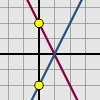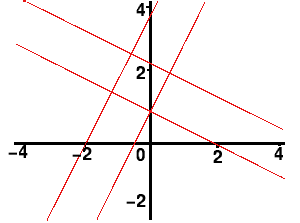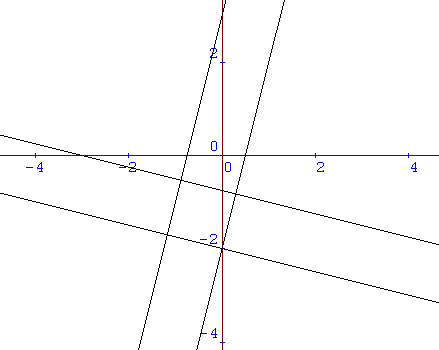#### You may also like### Parallel Lines

How does the position of the line affect the equation of the line? What can you say about the equations of parallel lines?### Diamond Collector

Collect as many diamonds as you can by drawing three straight lines.### Reflecting Lines

Investigate what happens to the equations of different lines when you reflect them in one of the axes. Try to predict what will happen. Explain your findings.

# Enclosing Squares

##### Age 11 to 14Challenge Level

We have had two good answers to this problem; one from Harry from Riccarton High School in Christchurch, New Zealand, and one from Ang Zhi Ping from River Valley High School in Singapore.

Harry starts by mentioning that there are two pairs of parallel lines, that the distance between each pair of lines has to be equal and that we need to have four right angles to make a square.

The gradient of the second pair of parallel lines is going to be the negative reciprocal of the first set of parallel lines in order to make right angles (ie. the product of their gradients will be -1).

For example:

2 -1/2
4 -1/4
-10 1/10

To keep the distance between each pair of lines the same, we'd have to make sure that the difference of the two y-intercepts of one pair of lines is equal to the difference of the two x-intercepts of the other pair.

In the example given in the question:
$y = 2x + 1$ and $y = 2x + 4$ cut the vertical axis at $(0,1)$ and $(0,4)$, three units apart,
and $y = -0.5x + 1$ and $y = -0.5x + 2.5$ cut the horizontal axis at $(2,0)$ and $(5,0)$, also three units apart.

 \begin{eqnarray} y &=& 2x + 1\\ y &=& 2x + 4 \\ y &=& -0.5x + 1\\ y &=& -0.5x + 2.5 \end{eqnarray}Alternatively, the difference of the y-intercepts of the second pair have to be the same as the difference of the $x$-intercepts of the first pair.

In the example given in the question:
$y = 2x + 1$ and $y = 2x + 4$ cut the horizontal axis at $(-0.5,0)$ and $(-2,0)$, one and a half units apart, and $y = -0.5x + 1$ and $y = -0.5x + 2.5$ cut the vertical axis at $(0,1)$ and $(0,2.5)$, also one and a half units apart.

This distance can be worked out easily:
it is the difference of the $y$-intercepts of the first pair divided by the gradient.

In the example above, the difference between the $y$-intercepts of $y = 2x + 1$ and $y = 2x + 4$ is three. This difference divided by the gradient of two gives us $1.5$; this is the difference between the $y$-intercepts of the other pair of lines, $y = -0.5x + 1$ and $y = -0.5x + 2.5$.

Consider another example:
If you are given the equations of two parallel lines
$y = 4x - 2$ and $y = 4x + 3$
and told that one of the vertices of the squares will be at $(0,-2)$
we can deduce that the equation of one of the other lines will be
$y = -0.25x - 2$ (perpendicular to the original pair with an intercept at $-2$).
Since the difference between the y-intercepts of $y = 4x - 2$ and $y = 4x + 3$ is five,
the difference between the y-intercepts of the other pair of lines will be five divided by the gradient ($5/4$ or $1.25$).
One of the perpendicular lines is $y = -0.25x - 2$, so the other line can be at $y = -0.25x - 0.75$,
cutting the vertical axis $1.25$ units above the first line.

 \begin{eqnarray} y &=& 4x -2\\ y &=& 4x + 3 \\ y &=& -0.25x -2\\ y &=& -0.25x -0.75 \end{eqnarray}By following the rules above, you could get the following formula:
If you are given the equations of two parallel lines $y = ax + b$ and $y = ax + c$ and you know that one of the vertices is at $(0,b)$ the equations of the other two lines will be:
$y = (-1/a)x + b$ and $y = (-1/a)x + b + (c - b)/a$
[$(c - b)/a$ is the difference between the intercepts of the first pair of lines divided by their gradient.]

For example: $y = 2x + 2, y = 2x + 6, y = -0.5x + 2, y = -0.5x + 4$.

Ang Zhi Ping noticed that the 4th line could lie either above or below $y = (-1/a)x + b$.

Thus, in order to make the solution complete, we should say that the two possible equations for the 4th line are: $$y = ({-1\over a})x +b \pm {(c-b)\over a}.$$